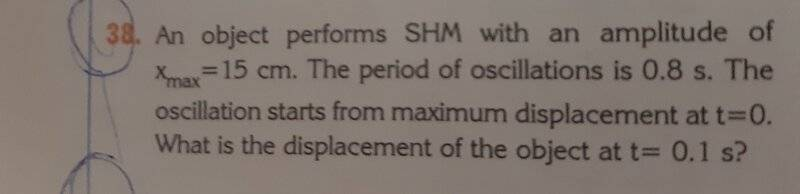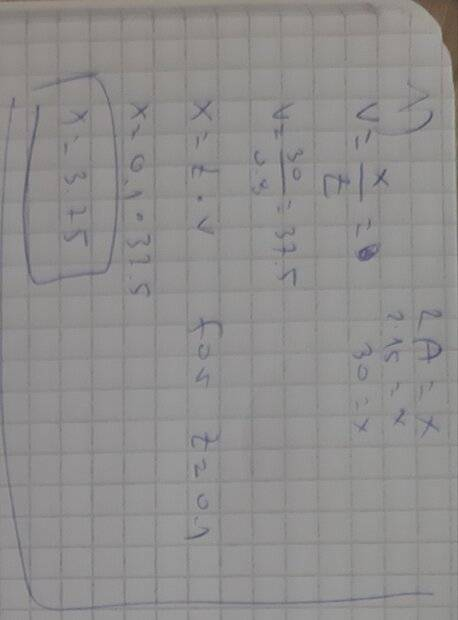# The 38th problem: I'm not getting the result in the book (SHM problem)

Pawn
Homework Statement:
The 38 problem:The result on the book is 10.6cm i keep getting 3.75
Relevant Equations:
X(t)=A×cos(w×t)Last edited:

## Answers and Replies

Homework Helper
Gold Member
2021 Award
Please show your work so that we can figure out where you went astray.

•topsquark
Pawn
Please show your work so that we can figure out where you went astray.

Please show your work so that we can figure out where you went astray.Gold Member
Type your work in Latex

Homework Helper
Gold Member
2021 Award
You are assuming that SHM is motion under zero acceleration. It is not. The velocity in SHM is not related to position and time by ##v=\frac{x}{t}##. What are the correct expressions for position and velocity in SHM? Your textbook should have those.

And yes, please learn how to write equations in LaTeX. Meanwhile, please do no post pictures sideways.

•MatinSAR
Gold Member
How can you get a displacement of 37.5 if the max amplitude is specified as 15?
(This is intended to inspire a sanity check. If you mentally draw this motion in your head, that may help you figure out where you're going wrong.)

•MatinSAR
Pawn
You are assuming that SHM is motion under zero acceleration. It is not. The velocity in SHM is not related to position and time by ##v=\frac{x}{t}##. What are the correct expressions for position and velocity in SHM? Your textbook should have those.

And yes, please learn how to write equations in LaTeX. Meanwhile, please do no post pictures sideways.
The only other equation i have is the one for the velocity in an instant of time v(t)=-vmax × sin(w × t).

W-omega
And i dont know what LaTeX is.

Pawn
How can you get a displacement of 37.5 if the max amplitude is specified as 15?
(This is intended to inspire a sanity check. If you mentally draw this motion in your head, that may help you figure out where you're going wrong.)
3.75*

Gold Member
3.75*
mm OK. Your notes say 3.75, though your problem statement says 37.5.
Not sure if you can still edit post #1.

Homework Helper
Gold Member
2021 Award
The only other equation i have is the one for the velocity in an instant of time v(t)=-vmax × sin(w × t).

W-omega
And i dont know what LaTeX is.
If your textbook really does not have an expression for the position as a function of time, look it up on the web.

To find how to use LaTeX to write and post equations, go here
https://www.physicsforums.com/help/latexhelp/
or click the link LaTeX Guide, lower left corner.

Pawn
If your textbook really does not have an expression for the position as a function of time, look it up on the web.

To find how to use LaTeX to write and post equations, go here
https://www.physicsforums.com/help/latexhelp/
or click the link LaTeX Guide, lower left corner.
Do you have any idea on how to solve it?

Homework Helper
Knowing the period allows you to figure out ω. Knowing the position at t=0 allows you to figure out A. Then obtain x(t) for t=0.1s.

Pawn
Knowing the period allows you to figure out ω. Knowing the position at t=0 allows you to figure out A. Then obtain x(t) for t=0.1s.
How ?

Homework Helper
Look in your book for the relation between T and ω. Tell me what it is

Pawn
Look in your book for the relation between T and ω. Tell me what it is
ω=2π/T

Homework Helper
So in your equation for x $$x(t)=A\cos\frac {2\pi t} T$$ Now what is A?

Pawn
A is 15cm. Right?

•hutchphd
Homework Helper
so t/T is 1/8 for t=0.1s

Pawn
So in your equation for x $$x(t)=A\cos\frac {2\pi t} T$$ Now what is A?
But if we substitute the values we dont get the result that is in the book.

Homework Helper
Gold Member
2021 Award
Do you have any idea on how to solve it?
I have lots of ideas how to solve it, but I will back off and let @hutchphd take over. You're in good hands.

•Pawn
Homework Helper
cos (pi/4)= ? ( thats 45deg)

Pawn
cos (pi/4)= ?
Do we substitute pi with the value 3.14

Homework Helper
You prefer 2pi/8 ??? see #18

Pawn
You prefer 2pi/8 ??? see #18
No sorry i didnt realise it

Pawn
cos (pi/4)= ? ( thats 45deg)
0.70

•